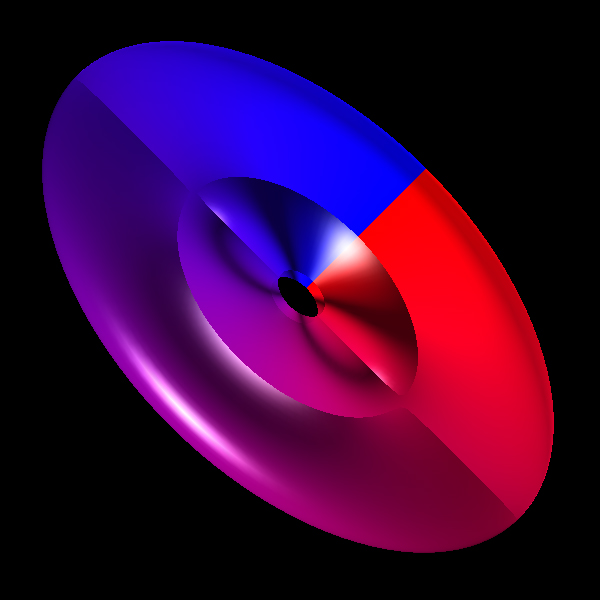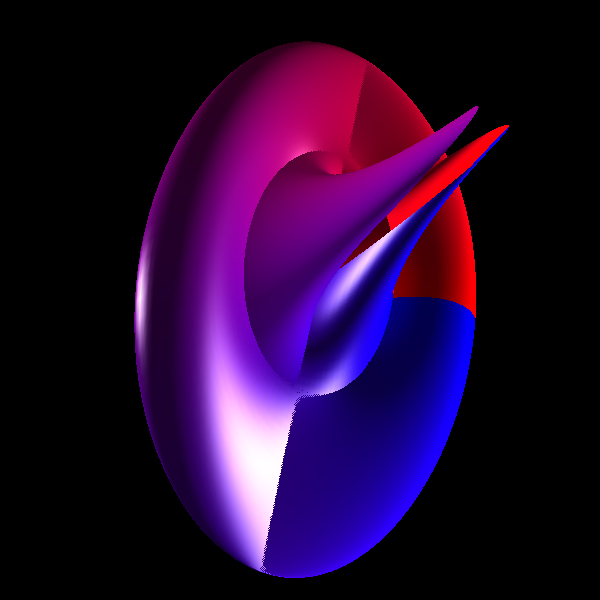# Folium

Contributed by Roger Bagula
Graphics by Paul Bourke
May 2003
x = cos(u) (2 v/ pi - tanh(v))
y = cos(u + 2 pi / 3) / cosh(v)
z = cos(u - 2 pi / 3) / cosh(v)
-pi <= u <= pi
-pi <= v <= pi